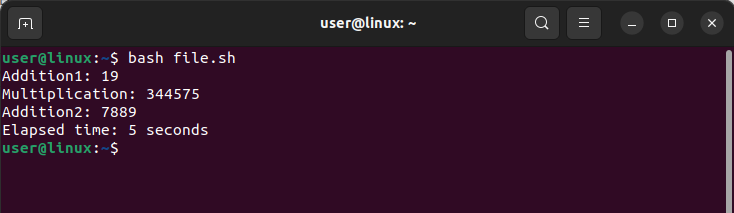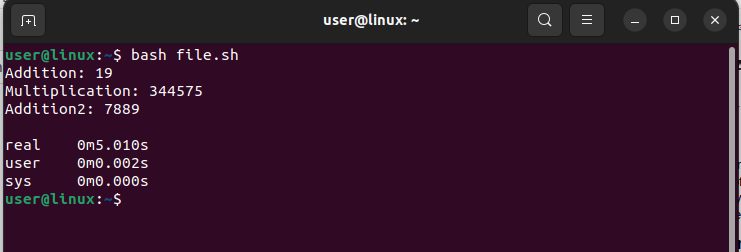# How to Measure Elapsed Time in Bash

Measurement of the elapsed time in a bash script is a useful tool for learning about performance, resource usage, and task execution times. Understanding how to quantify elapsed time can be incredibly useful for automated testing, performance analysis, monitoring, and troubleshooting. This post will examine various techniques for calculating elapsed time in a bash script, giving you the tools to increase your script’s effectiveness and optimize its performance and for demonstration Ubuntu operating system is used.

## How to Measure Elapsed Time in bash

There are various techniques for calculating elapsed time in a bash script, and each of them offers a clear-cut approach to calculate the time taken to complete a task:

• Through date command
• Through time command

### Through date Command

Another way to find the elapsed time in bash script is by using date command, with the ‘date’ command, you can retrieve the current date and time in a specified format, and store it in a variable, here is the syntax for it:

 `(date [Format])`

The Format string can include various codes for different elements of the date and time, such as %Y for the year, %m for the month, %d for the day of the month, %H for the hour, %M for the minute, and %S for the second.
To illustrate the use of this command and for educational purposes I have given an example code of bash script in which I have performed a simple task and find out the elapsed time using the above given syntax:

 ```#!/bin/bash start=\$(date +%s) Addition1=\$((10+9)) Multiplication=\$((385*895)) Addition2=\$((1100+6789)) sleep 5 end=\$(date +%s) elapsed=\$((end-start)) echo "Addition1: \$Addition1" echo "Multiplication: \$Multiplication" echo "Addition2: \$Addition2" echo "Elapsed time: \$elapsed seconds"```

Here is the output for the example code that demonstrate the use of date command to measure the elapsed time:Here, the code only executes addition, subtraction, multiplication, and five seconds of system sleep. I used the date command twice to determine the elapsed time: once to save the start time and once to save the end time. The elapsed time is then calculated by taking the difference between the two timings.

### Through time Command

The time command can be used to calculate the total amount of time taken by a script or a group of commands. It also provides other information, such as the amount of user and system CPU time, the amount of memory that can be used at maximum capacity, and the command’s exit status. Here is how to use this command in shell script to get the amount of time that has passed:

 `time `

I’ve included an example code of a bash script in which I’ve done a straightforward job and found the elapsed time using the above-given syntax to demonstrate how to use this command and for educational purposes:

 ```#!/bin/bash time { Addition1=\$((10+9)) Multiplication=\$((385*895)) Addition2=\$((1100+6789)) echo "Addition: \$Addition1" echo "Multiplication: \$Multiplication" echo "Addition2: \$Addition2" sleep 5 }```

The result for the example code that shows how to use the time command to calculate elapsed time is shown below:Here, it only executes four operations: addition, subtraction, and multiplication and puts the system to sleep for five seconds. The result indicates that the execution duration was almost five seconds.

## Conclusion

There are two ways to calculate the amount of time that has passed: using the ‘time’ command or the ‘date’ command. The ‘date’ command uses the start time and end time as starting points before calculating the difference to determine the amount of time that has passed. The ‘time’ command, which is a feature of the shell, can be used to calculate how long a command will take to execute.